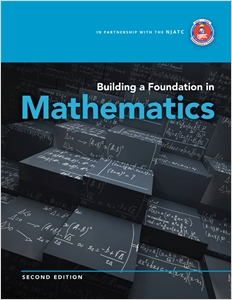I'm interested in (select all that apply)Building a Foundation in Mathematics

ISBN 13: 9781435488540

ISBN 10: 1435488547

Author: National Joint Apprenticeship Training Committee; John C. Peterson

Pages: 656

Published: 04/09/2010

Description
Benefits
• Expands student resources to include beginner-level electrical applications at the end of each chapter.
• Provides practical, easily applicable tools like the newly updated appendix depicting usage of a T1-30XII calculator.
• Packs content full of industry-specific examples and exercises that cater to the electronics field, offering a learning experience that is contextually meaningful and relevant.
• Reinforces key concepts with the dynamic use of color and detailed graphics that provide useful visualization aids.
• Facilitates the application of subject matter beyond confines of the book with handy appendices that include a guide to mathematical symbols, common percent, fraction, and decimal equivalents, geometry formulas, and more.1. Whole Numbers.
Writing and Rounding Whole Numbers. Adding Whole Numbers. Subtracting Whole Numbers. Multiplying Whole Numbers. Dividing Whole Numbers.
2. Fractions.
Writing and Ordering Fractions. Converting Improper Fractions and Mixed Numbers. Adding Fractions. Subtracting Fractions. Multiplying Fractions and Mixed Numbers. Dividing Fractions and Mixed Numbers. Reading a Customary Rule.
3. Decimals.
Writing and Rounding Decimals. Adding Decimals. Subtracting Decimals. Multiplying Decimals. Dividing Decimals. Converting Decimals and Fractions. Reading a Metric Rule.
4. Integers.
Introducing Integers. Adding Integers. Subtracting Integers.
Multiplying Integers. Dividing Integers.
5. Rational Numbers.
Adding Rational Numbers. Subtracting Rational Numbers. Multiplying Rational Numbers. Dividing Rational Numbers.
6. Exponents.
Introducing Exponents. Applying Laws of Exponents. Using Scientific Notation. Simplifying Expressions with Powers of Ten. Using Engineering Notation. Introducing Roots and Fractional. Exponents.
7.Logarithms.
Finding Logarithms. Properties of Logarithms. Using Logarithms. Natural Logarithms. Applying Logarithms.
8. Units and Measurements.
Converting Units in the Customary System. Converting Units in the Metric System. Converting between Customary and Metric Units. Converting Measures of Temperature. Converting Measures of Area and Volume. Using the Mil.
9. Algebra Essentials.
Introducing Algebra. Using Properties of Addition and Multiplication. Simplifying Algebraic Expressions.
10. Equations, Formulas,and Inequalities.
Writing Equations. Solving One-Step Equations. Solving Multi-Step Equations. Using Formulas. Using Equations to Solve Word Problems. Writing Inequalities. Solving Inequalities.
11. Ratios, Rates, and Proportions.
Writing and Simplifying Ratios. Writing Unit Rates. Identifying and Solving Proportions. Solving Direct Proportion Problems. Solving Inverse Proportion Problems.
12. Percents.
Converting Fractions, Decimals, and Percents. Solving Percent Problems with Proportions. Solving Percent Problems with Equations. Solving Word Problems.
13. The Cartesian Plane.
Plotting Points on a Coordinate Plane. Graphing Linear Equations Using a Table of Values. Graphing Linear Equations Using Intercepts. Finding the Slope of a Line. Graphing Linear Equations Using Slope-Intercept Form. Writing Equations of Lines.
14. Systems of Equations.
Solving Systems of Equations by Graphing. Solving Systems of Equations by Substitution. Solving Systems of Equations by Elimination. Solving Systems of Equations for Three Unknowns. Using Systems to Solve Word Problems.
15. Plane Geometry.
Basic Concepts. Measuring and Drawing Angles. Angles, Angle Pairs, and Parallel Lines. Polygons and Their Properties. Finding Perimeter. Finding Area. Finding Circumference and Area of Circles. Cong...